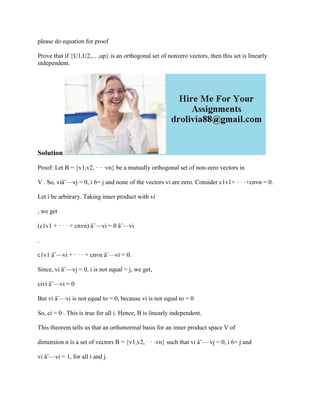Diese Präsentation wurde erfolgreich gemeldet.

# please do equation for proof Prove that if {U1-U2---- -up} is an ortho.docx

Anzeige
Anzeige
Anzeige
Anzeige
Anzeige
Anzeige
Anzeige
Anzeige
Anzeige
Anzeige
Anzeige×

1 von 2 Anzeige

# please do equation for proof Prove that if {U1-U2---- -up} is an ortho.docx

Prove that if {U1,U2,... ,up} is an orthogonal set of nonzero vectors, then this set is linearly independent.
Solution
Proof: Let B = {v1,v2, · · ·vn} be a mutually orthogonal set of non-zero vectors in
V . So, viâˆ—vj = 0, i 6= j and none of the vectors vi are zero. Consider c1v1+ · · ·+cnvn = 0.
Let i be arbitrary. Taking inner product with vi
, we get
(c1v1 + · · · + cnvn) âˆ—vi = 0 âˆ—vi
.
c1v1 âˆ—vi + · · · + cnvn âˆ—vi = 0.
Since, vi âˆ—vj = 0, i is not equal = j, we get,
civi âˆ—vi = 0
But vi âˆ—vi is not equal to = 0, because vi is not equal to = 0
So, ci = 0 . This is true for all i. Hence, B is linearly independent.
This theorem tells us that an orthonormal basis for an inner product space V of
dimension n is a set of vectors B = {v1,v2, · · ·vn} such that vi âˆ— vj = 0, i 6= j and
vi âˆ—vi = 1, for all i and j.
.

Prove that if {U1,U2,... ,up} is an orthogonal set of nonzero vectors, then this set is linearly independent.
Solution
Proof: Let B = {v1,v2, · · ·vn} be a mutually orthogonal set of non-zero vectors in
V . So, viâˆ—vj = 0, i 6= j and none of the vectors vi are zero. Consider c1v1+ · · ·+cnvn = 0.
Let i be arbitrary. Taking inner product with vi
, we get
(c1v1 + · · · + cnvn) âˆ—vi = 0 âˆ—vi
.
c1v1 âˆ—vi + · · · + cnvn âˆ—vi = 0.
Since, vi âˆ—vj = 0, i is not equal = j, we get,
civi âˆ—vi = 0
But vi âˆ—vi is not equal to = 0, because vi is not equal to = 0
So, ci = 0 . This is true for all i. Hence, B is linearly independent.
This theorem tells us that an orthonormal basis for an inner product space V of
dimension n is a set of vectors B = {v1,v2, · · ·vn} such that vi âˆ— vj = 0, i 6= j and
vi âˆ—vi = 1, for all i and j.
.

Anzeige
Anzeige

### please do equation for proof Prove that if {U1-U2---- -up} is an ortho.docx

1. 1. please do equation for proof Prove that if {U1,U2,... ,up} is an orthogonal set of nonzero vectors, then this set is linearly independent. Solution Proof: Let B = {v1,v2, · · ·vn} be a mutually orthogonal set of non-zero vectors in V . So, viâˆ—vj = 0, i 6= j and none of the vectors vi are zero. Consider c1v1+ · · ·+cnvn = 0. Let i be arbitrary. Taking inner product with vi , we get (c1v1 + · · · + cnvn) âˆ—vi = 0 âˆ—vi . c1v1 âˆ—vi + · · · + cnvn âˆ—vi = 0. Since, vi âˆ—vj = 0, i is not equal = j, we get, civi âˆ—vi = 0 But vi âˆ—vi is not equal to = 0, because vi is not equal to = 0 So, ci = 0 . This is true for all i. Hence, B is linearly independent. This theorem tells us that an orthonormal basis for an inner product space V of dimension n is a set of vectors B = {v1,v2, · · ·vn} such that vi âˆ— vj = 0, i 6= j and vi âˆ—vi = 1, for all i and j.